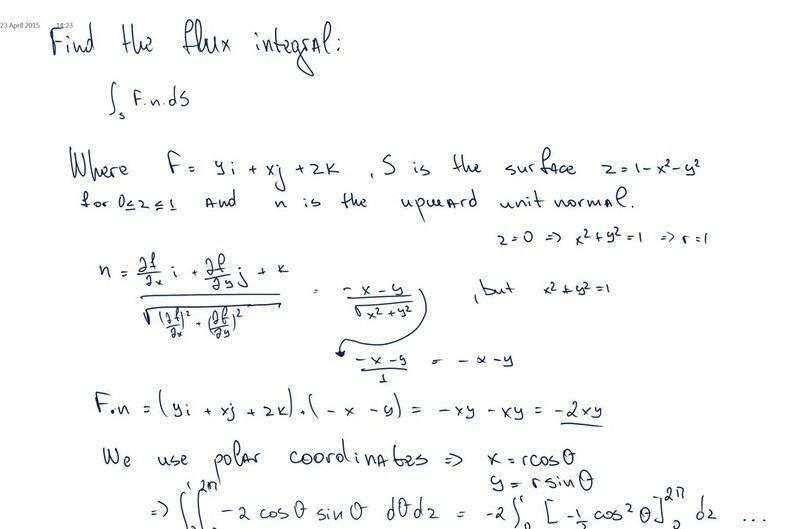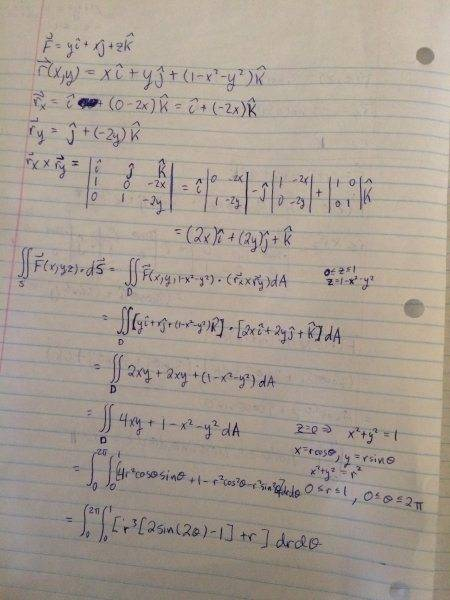# Flux Integral Problem

## Homework Statement

Hello,
So I've got to evaluate a flux integral, but I get 0. Could someone please, check my workings and give me advice.
Please find my workings and the problem attached here as an image.
Thank you very much!LCKurtz
Homework Helper
Gold Member
I will give you that your handwriting is pretty nice. Still it would be better to type your work. Is that third component of your flux vector a ##z## or a ##2##? Some mathematical issues are: Is your normal directed upward like it is supposed to be? In fact, is it even a vector? That's another reason to type your question, so we can edit it. Also you can't say ##x^2+y^2=1## for the normal. That is only true on the boundary of the surface but not on the surface itself. And check your partial derivatives.

STEMucator
Homework Helper
You can parametrize the surface if you want, let:

$$\vec r(x, y) = x \hat i + y \hat j + z \hat k = x \hat i + y \hat j + (1 - x^2 - y^2) \hat k$$

Find ##\vec r_x(x, y)## and ##\vec r_y(x, y)##. Use those to obtain ##\vec r_x \times \vec r_y##. Now you have a theorem which states:

$$\iint_S \vec F(x, y, z) \cdot d \vec S = \iint_D \vec F(\vec r(x,y)) \cdot (\vec r_x \times \vec r_y) \space dA$$

Where the region ##D## is given by the plane ##z = 1 - x^2 - y^2## for ##0 \leq z \leq 1##.

Plotting the plane for the points ##(x, 0, 0), (0, y, 0)##, and ##(0, 0, z)## will give you the limits easily, and a nice graph to look at.

LCKurtz
Homework Helper
Gold Member
You can parametrize the surface if you want, let:

$$\vec r(x, y) = x \hat i + y \hat j + z \hat k = x \hat i + y \hat j + (1 - x^2 - y^2) \hat k$$

Find ##\vec r_x(x, y)## and ##\vec r_y(x, y)##. Use those to obtain ##\vec r_x \times \vec r_y##. Now you have a theorem which states:

$$\iint_S \vec F(x, y, z) \cdot d \vec S = \color{red}{\pm}\iint_D \vec F(\vec r(x,y)) \cdot (\vec r_x \times \vec r_y) \space dA$$

Where the region ##D## is given by the plane ##z = 1 - x^2 - y^2## for ##0 \leq z \leq 1##.

Plotting the plane for the points ##(x, 0, 0), (0, y, 0)##, and ##(0, 0, z)## will give you the limits easily, and a nice graph to look at.

The ##\pm## sign is necessary because one has to decide whether ##\vec r_x \times \vec r_y## points in the required direction to agree with the given orientation. The OP didn't check that.

Got confused..

g = x2 + y2 + z -1 = 0
n = $\frac{ \nabla g}{|\nabla g|}$
n =$\frac{ 2x+2y+1}{\sqrt{2x^2+2y^2+1^2}}$
and dS =$\sqrt{2x^2+2y^2+1^2}$ dxdy
?

Last edited:
HallsofIvy
Homework Helper
g = x2 + y2 + z -1 = 0
n = $\frac{ \nabla g}{|\nabla g|}$
n =$\frac{ 2x+2y+1}{\sqrt{2x2+2y2 + 12}}$
This last is wrong. n is a vector not a scalar. What is $\nabla g$?

g(x,y,z) = z - f(x,y) = 0 according to my lecture notes.
I tried out the latex output, since the preview didn't work out. I've made other edits.
I am quite confused, I solved other questions using the method with g, but this one, don't know if it should be solved with rdrdθ or just dxdy . The confusing thing is that here I have z = 1-x2-y2

Last edited:
You can parametrize the surface if you want, let:

$$\vec r(x, y) = x \hat i + y \hat j + z \hat k = x \hat i + y \hat j + (1 - x^2 - y^2) \hat k$$

Find ##\vec r_x(x, y)## and ##\vec r_y(x, y)##. Use those to obtain ##\vec r_x \times \vec r_y##. Now you have a theorem which states:

$$\iint_S \vec F(x, y, z) \cdot d \vec S = \iint_D \vec F(\vec r(x,y)) \cdot (\vec r_x \times \vec r_y) \space dA$$

Where the region ##D## is given by the plane ##z = 1 - x^2 - y^2## for ##0 \leq z \leq 1##.

Plotting the plane for the points ##(x, 0, 0), (0, y, 0)##, and ##(0, 0, z)## will give you the limits easily, and a nice graph to look at.

Hello, I've got exam on the same things, so please give some feedback on my workings

This surface is the graph of the function $f(x,y) = 1-x^2-y^2 \mbox{ for } x^2+y^2 <= 1$, so use the graph parametrization
$X(x,y) = (y,x, 1 - x^2 - y^2).$
We shall use the following (very important!) general formula for the upward normal of a surface defined by an equation of the form $z = f(x,y):$
$N(x,y)=(-f1, -f2,1)$

For the surface of interest here, it follows that:
$N(x,y) = (-(-2x),-(-2y),1)$

And we have $F(X(x,y)) = (y,x,1-x^2-y^2)$

So $F \cdot N = 2xy+2xy+1-x^2 - y^2$

So we have $\ \int_0^{2\pi} \int_0^1 (4cos( \theta )sin( \theta ) + 1 - r^2) rdrd \theta$

STEMucator
Homework Helper
Sorry for that a bit earlier where I mentioned ##z = 1 - x^2 - y^2## is a plane, it's a paraboloid.

As for the actual question, I worked it out to be:Sorry for the squeeze near the bottom I was writing a bit too quickly and it got cut off.

Sorry for that a bit earlier where I mentioned ##z = 1 - x^2 - y^2## is a plane, it's a paraboloid.

As for the actual question, I worked it out to be:

View attachment 83290

Sorry for the squeeze near the bottom I was writing a bit too quickly and it got cut off.
Yeah, I got the same, I just played it smart, but I think your solution is correct. I substituted r = x + y in the integral ## 1 - x^2 - y^2## with ## 1 - ( x^2 + y^2)## to get ## 1 - r^2## and also for x and y since r = 1 I think I saw somewhere to replace ##rcos( \theta ) ## with ## 1cos( \theta )##
Otherwise your should be the correct one.